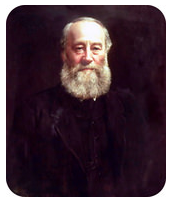# 1.6.4: SI Kinetic Energy Units

When a cat is chasing something, its moves very fast. A person may comment, "that cat has a lot of energy"—which is more correct than they might realize! One form of energy is seen when an object is moving, and this type of energy is the basis for many chemical processes.

## SI Kinetic Energy Units

An object's kinetic energy is the energy due to motion. Kinetic energy can be defined mathematically as:

$KE = \frac{1}{2} mv^2$

where $$KE$$ is kinetic energy, $$m$$ is mass, and $$v$$ is velocity.

Energy is defined as the capacity to do work or to produce heat. As discussed previously, kinetic energy is one type of energy and is associated with motion. Another frequently encountered energy is potential energy, a type of energy that is stored in matter and released during a chemical reaction. The joule $$\left( \text{J} \right)$$ is the SI unit of energy and is named after English physicist James Prescott Joule (1818-1889). If we go back to the equation for kinetic energy written above, we can put units in: $$\text{kg}$$ for mass and $$\text{m}^2/\text{s}^2$$ for velocity squared. Then, in terms of SI base units, a joule is equal to a kilogram times meter squared, divided by a second squared $$\left( \text{kg} \cdot \text{m}^2/\text{s}^2 \right)$$. Another common unit of energy that is often used is the calorie $$\left( \text{cal} \right)$$, which is equivalent to $$4.184 \: \text{J}$$.James Prescott Joule.

## Summary

• Energy is the capacity to do work or to produce heat.
• Kinetic energy is energy due to motion.
• Potential energy is energy stored in matter.
• The joule $$\left( \text{J} \right)$$ is the SI unit of energy and equals $$\text{kg} \cdot \text{m}^2/\text{s}^2$$.